﻿Commodity Channel Index

# Commodity Channel Index

First the Commodity Channel Index (Cci) was developed as the indicator for determining of reversal points in the commodity markets. But then it became rather popular in the share market. The supposition on which the indicator is based consists that all actives move under influence of definite cycles, and maxima and minima appear with definite intervals. Cci corresponds to oscillators, measuring speed of price fluctuations. The index demonstrates a deflection of the ongoing price from its average value.Market Signals

Commodity Channel Index usually varies in the range from -100 to 100. Values above 100 inform about overbuying state (and about a probability of correcting decay), and the values below -100 inform about the overselling state (and about a probability of correcting increase).Calculation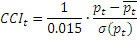where: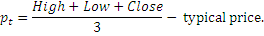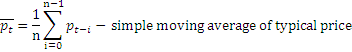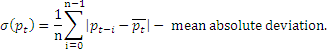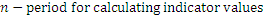Chart Example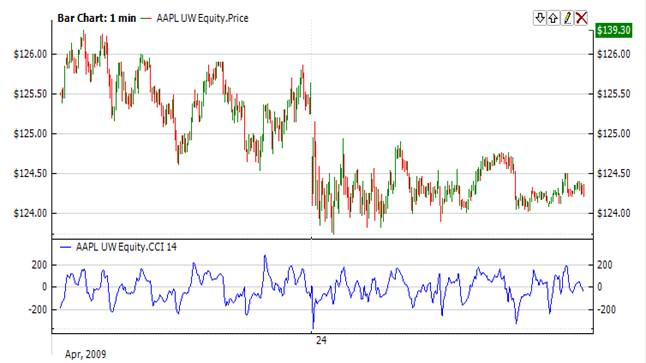Implementation and Usage

To initialize Cci use one of the following constructors:

Cci – set default values: period = 14

Cci(Int32) – set values for period

Cci(TimeSpan) – set time period

Use

CCI - property to get current valueExample
C#
``` 1// Create new instance
2Cci cci = new Cci(28);
3
4// Number of stored values
5cci.HistoryCapacity = 2;
6
8cci.Add(Bars.Current.Open, Bars.Current.High, Bars.Current.Low, Bars.Current.Close, Bars.Current.Volume, CurrentTime);
9
10// Get indicator value
11double IndicatorValue = cci.CCI;
12// Get previous value
13if (cci.HistoryCount == 2)
14{
15    double IndicatorPrevValue = cci;
16}```

Checks whether Cci crosses the 100 line:

C#
``` 1using FinAnalysis.TA;
2using FinAnalysis.Base;
3
4Cci cci;
5public override void OnInit()
6{
7    //...
8    // Create new instance, CCI period = 14
9    cci = new Cci(14);
10    // Store 2 indicator values
11    cci.HistoryCapacity = 2;
12    //...
13}
14
15public override void OnBarClose()
16{
17    //...
19    if (cci.HistoryCount >= 2)
20    {
21        if (cci.CCI > 100 && cci <= 100)
22        {
23            // Cross logic
24        }
25    }
26    //...
27}```

Note that the expressions

C#
`1double IndicatorValue = cci;`

and

C#
`1double IndicatorValue = cci.CCI`

are equivalent, but in the first case you must specify HistoryCapacity property.